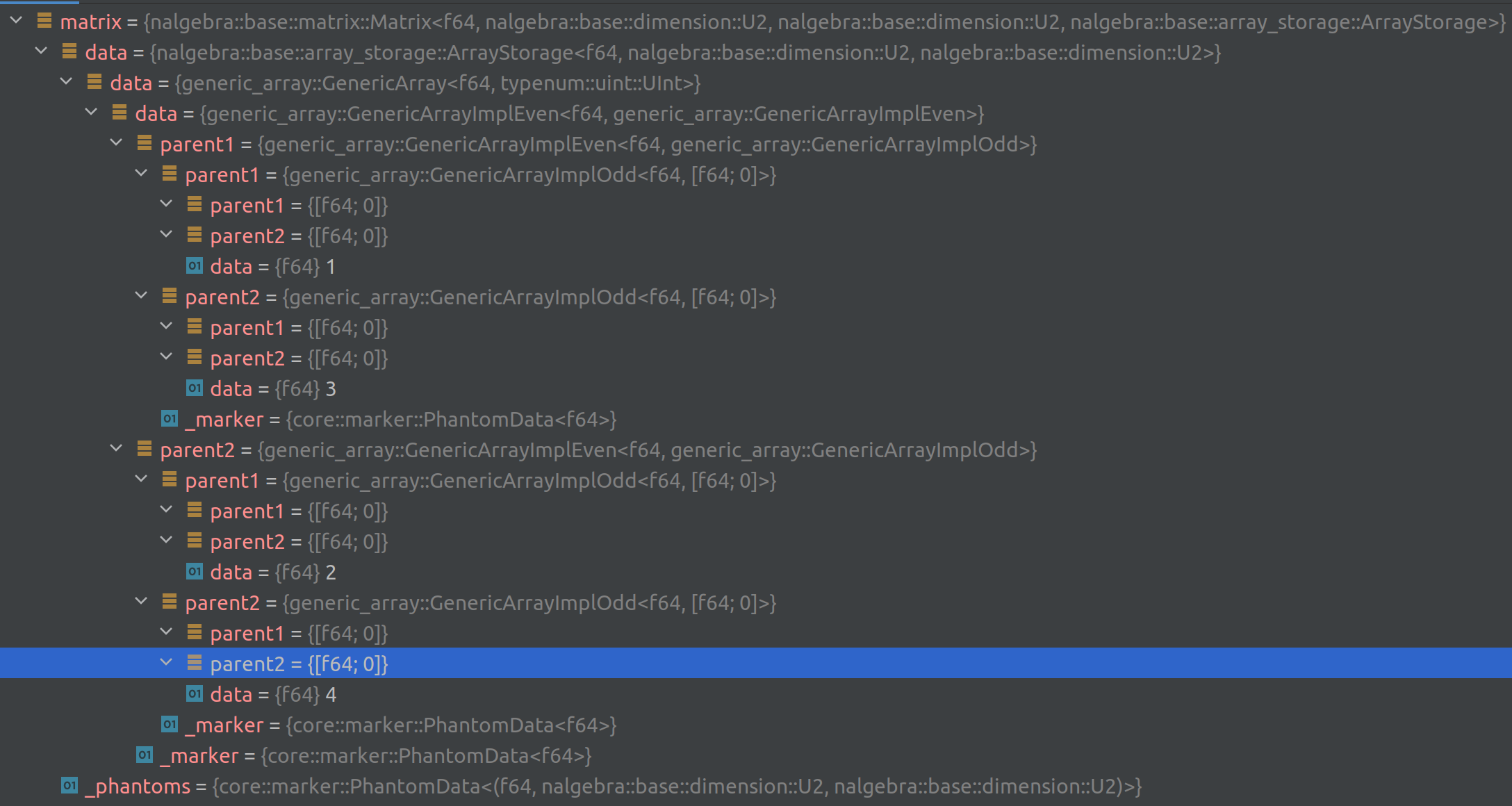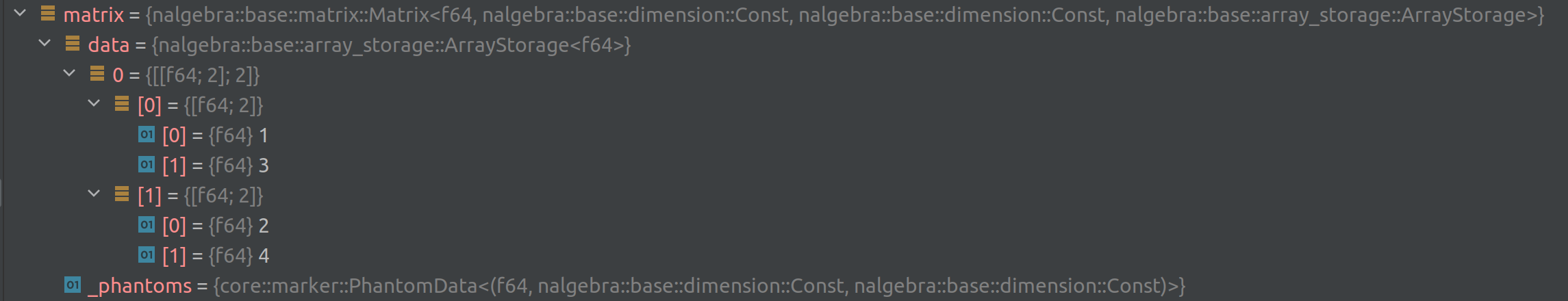# Integrating const-generics to nalgebra 0.26

Today we released the version 0.26 of our general-purpose linear-algebra crate: nalgebra 🎊

The version 1.51.0 of Rust has been released three weeks ago. That version stabilized an MVP for one of the feature we wanted the most: const-generics. Const-generics allow you to define types parametrized by const integers, chars, or booleans. One iconic example is writing a structure wrapping an array of any size:

// Example taken from the 1.51 Rust announcement.
struct Array<T, const LENGTH: usize> {
list: [T; LENGTH]
}

nalgebra supports matrices (and vectors) with dimensions known at runtime or at compile-time. The components of matrices/vectors with dimensions known at runtime are stored in a `Vec<T>`. Before nalgebra 0.26, matrices/vectors with dimensions known at compile-time were stored in a `GenericArray`, from the excellent generic-array crate. Thanks to const-generics, we were able to replace `GenericArray` by standard arrays: `[[T; R]; C]` (where `R` is the number of rows, and `C` the number of columns) in nalgebra 0.26.

This change results in significant ergonomics improvements when using statically-sized matrices or vectors:

### 1. Simpler generic programming with statically-sized entities#

Using nalgebra for generic programming with statically-sized matrices/vectors/points was quite challenging before. Let's take a simple example of a generic structure that wraps a point, a vector, and a matrix and performs some kind integration using 64-bit precision. In previous version of nalgebra this would look like this:

Before (nalgebra < 0.26)
use nalgebra::{DimName, MatrixMN, VectorN, Point, DefaultAllocator, allocator::Allocator};
struct Integrator<D: DimName>
where DefaultAllocator: Allocator<f32, D, D> + Allocator<f32, D> {
m: MatrixMN<f32, D, D>,
v: VectorN<f32, D>,
p: Point<f32, D>
}
impl<D: DimName> Integrator<D>
where DefaultAllocator: Allocator<f32, D, D> + Allocator<f32, D> {
// A method that performs some kind of integration
// using `f64` intermediate results.
pub fn integrate_highp(&self, dt: f32) -> Point<f32, D>
where
MatrixMN<f32, D, D>: Copy,
VectorN<f32, D>: Copy,
Point<f32, D>: Copy,
DefaultAllocator: Allocator<f64, D, D> + Allocator<f64, D> {
// First cast all the operants to `f64`:
let (m, v, p) = (self.m.cast::<f64>(), self.v.cast::<f64>(), self.p.cast::<f64>());
// Perform the computation with f64 precision, then
// cast the result to f32.
(p + m * v * dt as f64).cast()
}
}

See all the `DefaultAllocator: Allocator<...>` trait bounds? They are here to help the compiler deduce the proper storage types for the matrix/vector/points (i.e. to deduce the `GenericArray` with the right dimensions).

Now that const-generics have been integrated to nalgebra this code becomes as simple as one would expect:

Now (nalgebra 0.26)
use nalgebra::{Point, SMatrix, SVector};
struct Integrator<const D: usize> {
m: SMatrix<f32, D, D>,
v: SVector<f32, D>,
p: Point<f32, D>,
}
impl<const D: usize> Integrator<D> {
// A method that performs some kind of integration
// using `f64` intermediate results.
fn integrate_highp(&self, dt: f32) -> Point<f32, D> {
// First cast all the operants to `f64`:
let (m, v, p) = (
self.m.cast::<f64>(),
self.v.cast::<f64>(),
self.p.cast::<f64>(),
);
// Perform the computation with f64 precision, then
// cast the result to f32.
(p + m * v * dt as f64).cast()
}
}

See that there isn't any `DefaultAllocator: Allocator` trait bounds whatsoever.

Now, keep in mind that these simplifications will only work if you stick with entities with dimensions known at compile-time. If your code needs to work generically for both statically-sized matrices and dynamically-sized matrices, then the `DefaultAllocator: Allocator` bounds are still necessary (we should be able to get rid of them too once specialization is stabilized).

### 2. Simpler debugging of small matrices and vectors#

This one is a life-changer for those relying extensively on debuggers. Until now, inspecting with a debugger the content of statically-sized matrices or vectors (or points, quaternions, etc.) was extremely difficult. This was caused by the smart, but complicated, recursive definition of `GenericArray`. Here is what inspecting the content of `let matrix = Matrix2::new(1, 2, 3, 4)` looked like (in CLion's debugger, using the Rust plugin):Here is what it looks like now that we replaced `GenericArray` by standard arrays:This is much clearer now. Keep in mind that nalgebra stores its matrices in column-major format. That's why the components appear in the order `[[1, 3], [2, 4]]` (column by column).

### 3. Building small, constant, matrices and vectors#

Now that small matrices are using standard arrays under the hood, it is now possible to define constant vectors, matrices, points, quaternions, and translations by using their `const fn new(...)` constructors:

use nalgebra::{Vector3, Point4, Matrix2, Quaternion, Translation3};
const VECTOR: Vector3<u32> = Vector3::new(1, 2, 3);
const POINT: Point4<f32> = Point4::new(1.0, 2.0, 3.0, 4.0);
const MATRIX: Matrix2<i32> = Matrix2::new(-1, 2,
-3, 4);
const QUATERNION: Quaternion<f64> = Quaternion::new(1.0, 2.0, 3.0, 4.0);
const TRANSLATION: Translation3<f32> = Translation3::new(1.0, 2.0, 3.0);

### 4. New aliases#

Everything in nalgebra ends up being expressed as a matrix, in one way or another. Even a vector is just the `Matrix` type setup to have only one column. This is why we have so many type aliasses like `Vector3` which is an alias for a matrix with 3 rows and one column.

In nalgebra 0.26 we have the following dimension-generic type aliases:

• `OMatrix<T, R: Dim, C: Dim>`: for owned matrices (formerly called `MatrixMN`), i.e., matrices that own their components (as opposed to matrix slices that borrow them).
• `SMatrix<T, const R: usize, const C: usize>`: for statically-allocated matrices.
• `DMatrix<T>`: for dynamically-allocated matrices.

All the aliases for specific dimensions (e.g. `Vector3` for 3D vectors) remain unchanged.

The same applies to vectors: `OVector, SVector, DVector`. For example a statically-sized vector with 528 components can be written `SVector<f32, 528>`.

## Limitations: why `typenum` is still needed#

We no longer use the `generic-array` crate, but you will notice that nalgebra continues to depend on the `typenum` crate. This is needed because const-generics support on Rust 1.51 isn't complete yet, and doesn't allow operations on the const-parameters like in the following `push` method that adds an element to an array:

impl<T, const LENGTH: usize> Vector<T, LENGTH> {
// The { LENGTH + 1 } isn't allowed yet in this context.
fn push(self) -> Vector<T, { LENGTH + 1 }>
{ unimplemented!() }
}

In order to overcome this limitation, we have to rely on `typenum` by converting the integer to a type-level-integer, performing the operation with `typenum`'s type-level operators, and then extracting the result as a const value. This roughly looks like this:

struct Const<const D: usize>;
trait ToTypenum {
type Output;
}
trait ToConst {
type Output;
}
impl ToTypenum for Const<1> { type Output = typenum::U1; }
impl ToConst for typenum::U1 { type Output = Const<1>; }
impl ToTypenum for Const<2> { type Output = typenum::U2; }
impl ToConst for typenum::U2 { type Output = Const<2>; }
// ... make the same impl. for all values until some arbitrary
// limit (currently 127 in nalgebra).
impl<const R: usize> Vector<N, Const<R>> {
fn push(&self) -> Vector<N, <Sum<<Const<R> as ToTypenum>::Output, typenum::U1> as ToConst>::Output>
where Const<R>: ToTypenum,
Sum<<Const<R> as ToTypenum>::Output, typenum::U1>: ToConst {
unimplemented!()
}
}

It's actually less verbose in nalgebra because we have some additional traits to hide some of the complexity going on here. But the fact remains that without more advanced const-generics support from the compiler, methods like this `push` will be more verbose to define, and will be limited to the values of `D` (currently the values in `[0, 127]`) such that `Const<D>: TyTypenum` is implemented.

## What's next?#

The const-generics MVP allowed us to significantly improve the ergonomics of statically-sized matrices and vectors. However, we are still waiting for two features to land to make nalgebra much nicer:

• Specialization: with specialization in place, we could get rid of all the `DefaultAllocator: Allocator<...>` bounds. This means writing generic code that accepts both statically-sized matrices and dynamically-sized matrices would become much easier.
• Const-generics (the complete version): being able to write something like `[T; { LENGTH + 1 }]` would allow us to remove the `typenum` dependency completely.
• Const-fn (the complete version): being able to have trait bounds other than `Sized` in generic const-functions would allow us to mark most of nalgebra's methods as `const`. Right now, only some constructors are possible.